# Suit

The suit cost 119 euros. How much cost the pants if it is 50% cheaper than the jacket.

a =  39.6667 Eur

### Step-by-step explanation:

a+b = 119
a = (1-50/100) b

a+b = 119
a = (1-50/100)•b

a+b = 119
100a-50b = 0

a = 119/3 ≈ 39.666667
b = 238/3 ≈ 79.333333

Our linear equations calculator calculates it.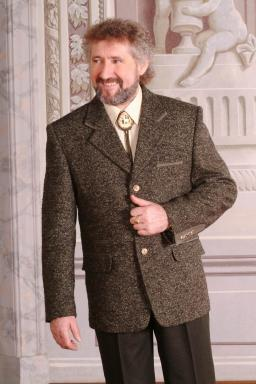Did you find an error or inaccuracy? Feel free to write us. Thank you!Tips to related online calculators
Check out our ratio calculator.
Do you have a linear equation or system of equations and looking for its solution? Or do you have a quadratic equation?

## Related math problems and questions:

• PaintingThe room is long 50 meters and wide 60dm and 300 cm high. Calculate how much it will cost painting if area of windows and doors is 15% of the total area. One square meter cost 50cents.
• Gasoline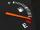For nearly full tank of 40 liters of gasoline chauffeur paid 54 euros. How many euros it will cost to go 250 km long if the car has an average fuel consumption 7 liters per 100 kilometers?
• Sales off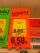shoes cost x euros. First they are discounted by 50%, and then 25% of the new amount. Determine what percentage of the total cost is cheaper.
• Product V2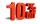The price of product was increased by 12%. Price increased by 273. How much money it would cost this product, if it was on the contrary by 19% discounted.
• Tape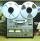Video is 153% more expensive than tape recorder. How many percent is tape recorder less expensive than video?
• The percentages in practiceIf every tenth apple on the tree is rotten it can be expressed by percentages: 10% of the apples on the tree is rotten. Tell percent using the following information: a. in June rained 6 days b, increase worker pay 500 euros to 50 euros c, grabbed 21 from
• Price saleoffShoes standing y euros. At first, they were discounted by 12% and then 50% of the new amount. After this double cheapening the cost was exactly 22 euros. Determine the original price of the shoes.
• Clothes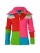CZK 2,400 cost clothes, a sweater by 150% than a T-shirt, trousers 2x more than a sweater, a jacket as a sweater and trousers together. What did the individual clothes cost?
• Unions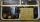Calculate how much money per year would go to the treasury of unions if 976 thousand employees join unions with an average net salary of 587 euros and if union membership cost 1% of the net monthly salary.
• A ball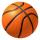A ball and a plush toy cost 32.80 euros. How much does a ball cost if it is € 9.50 cheaper than a toy?
• Brunette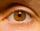Of the girls who attend primary school are 30% blondes and 70% brunette. 81% blondes and 19% brunette has blue eyes. How many girls attending school altogether if 470 girls has blue eyes?
• Sale off 2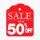A pair of of blues jeans went on sale. After a 30% reduction the pants cost \$35. How much did the jeans cost before the price reduction?
• Four pupilsFour pupils divided \$ 1485 so that the second received 50% less than the first, the third 1/2 less than a fourth, and fourth \$ 154 less than the first. How much money had each of them?
• The notebook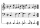After rising by 40%, the notebook cost 10.50 euros. How much did this notebook cost if it increased in price by only 20% instead of 40%?
• Washing machineThe price of washing machine was decreased by 21% and then by 55 € due small sales. After two price decreases cost € 343. How much was originally cost?
• VAT on booksThe cost of a book in the store is 12.5 euros. How much euros is the VAT of this book? VAT is 10%.
• 100 %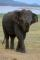How much is 100%, if 17 % is 1169?